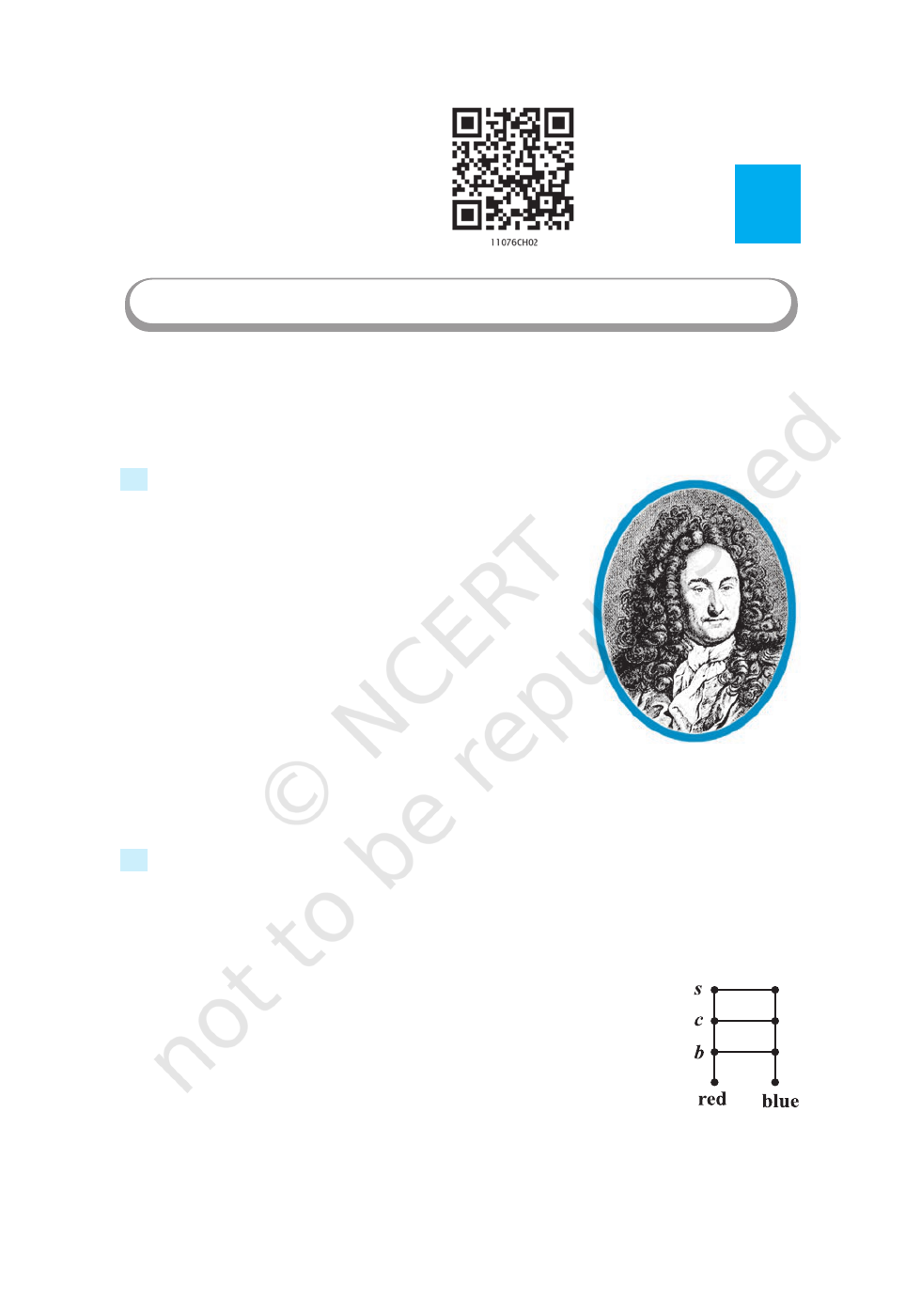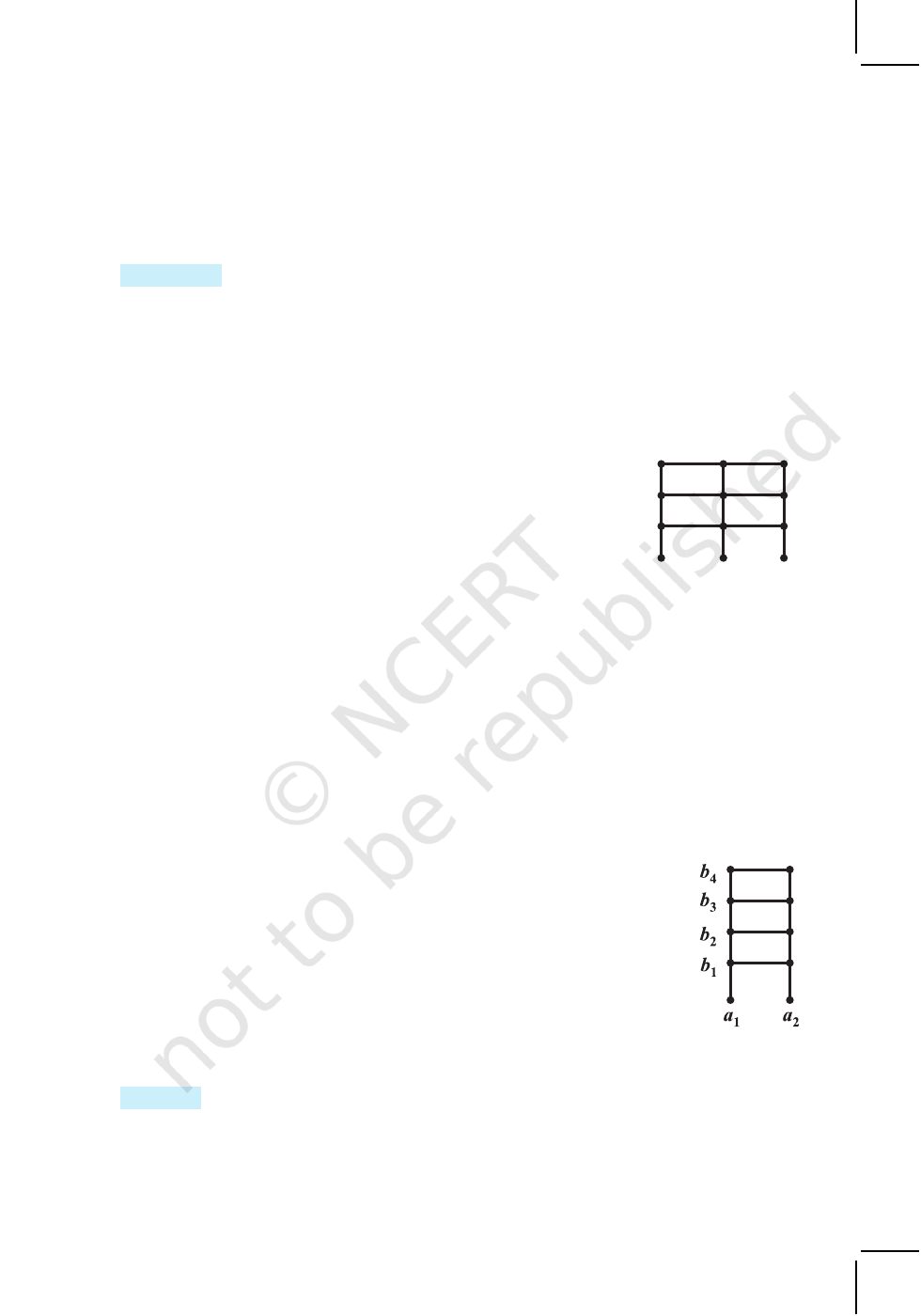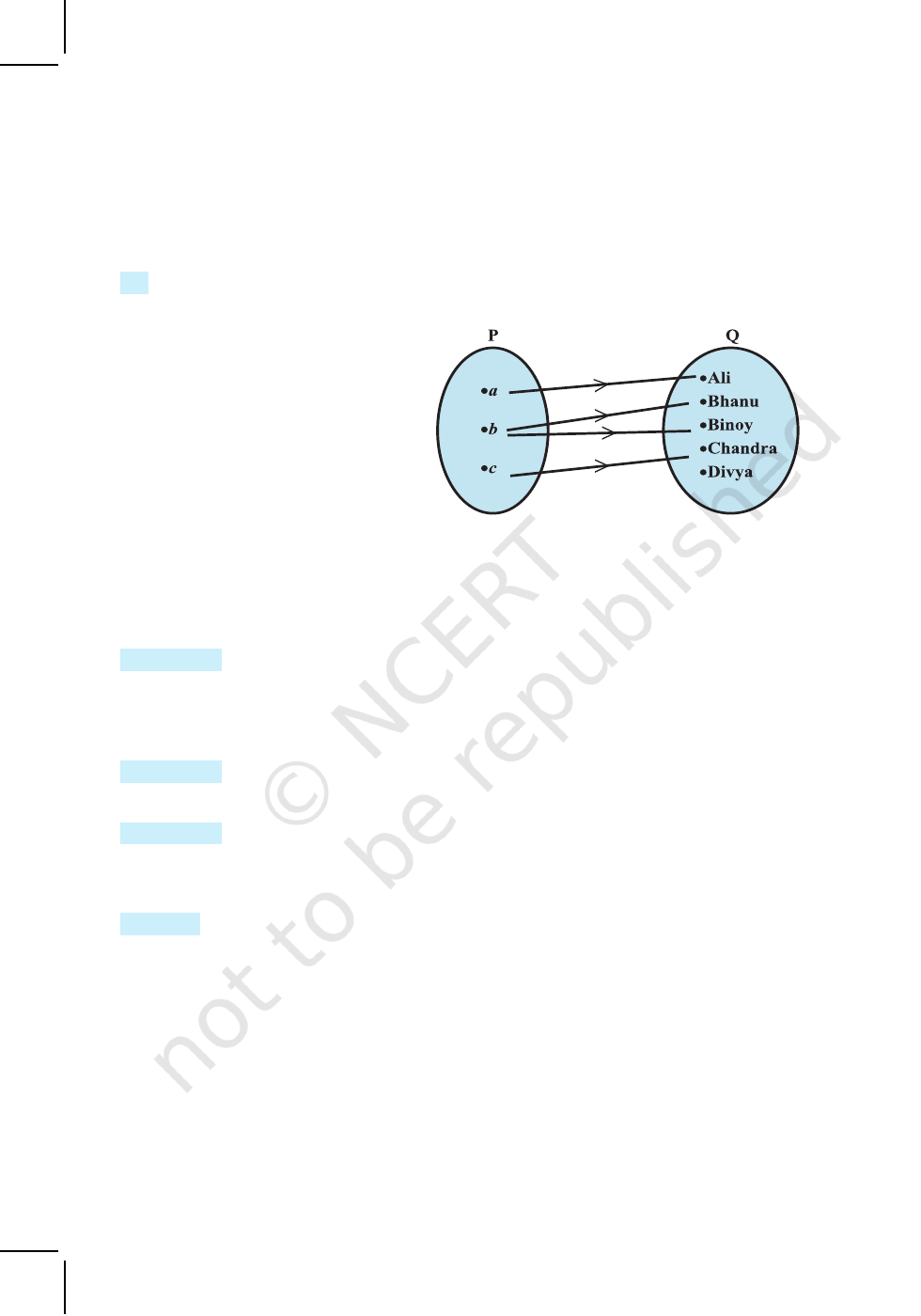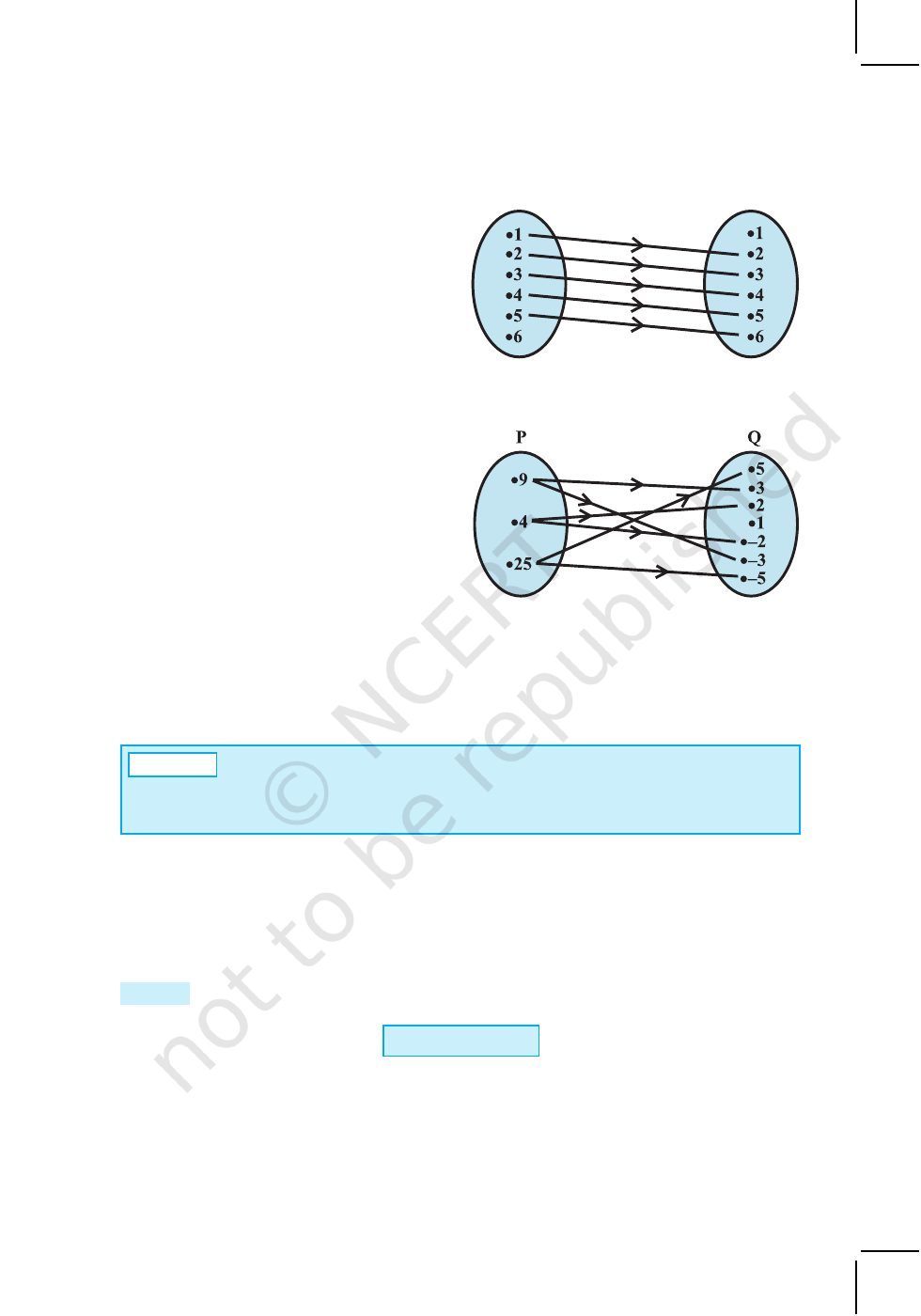vMathematics is the indispensable instrument of
all physical research. – BERTHELOT v
2.1 Introduction
Much of mathematics is about finding a pattern – a
recognisable link between quantities that change. In our
daily life, we come across many patterns that characterise
relations such as brother and sister, father and son, teacher
and student. In mathematics also, we come across many
relations such as number m is less than number n, line l is
parallel to line m, set A is a subset of set B. In all these, we
notice that a relation involves pairs of objects in certain
order. In this Chapter, we will learn how to link pairs of
objects from two sets and then introduce relations between
the two objects in the pair. Finally, we will learn about
special relations which will qualify to be functions. The
concept of function is very important in mathematics since it captures the idea of a
mathematically precise correspondence between one quantity with the other.
2.2 Cartesian Products of Sets
Suppose A is a set of 2 colours and B is a set of 3 objects, i.e.,
A = {red, blue}and B = {b, c, s},
where b, c and s represent a particular bag, coat and shirt, respectively.
How many pairs of coloured objects can be made from these two sets?
Proceeding in a very orderly manner, we can see that there will be 6
distinct pairs as given below:
(red, b), (red, c), (red, s), (blue, b), (blue, c), (blue,
s).
Thus, we get 6 distinct objects (Fig 2.1).
Let us recall from our earlier classes that an ordered pair of elements
taken from any two sets P and Q is a pair of elements written in small
Fig 2.1
Chapter
2
RELATIONS AND FUNCTIONS
G . W. Leibnitz
(1646–1716)
2020-21RELATIONS AND FUNCTIONS 31
brackets and grouped together in a particular order, i.e., (
p,q), p P and q Q .
This
Definition 1
Given two non-empty sets P and Q. The cartesian product P × Q is the
set of all ordered pairs of elements from P and Q, i.e.,
P
× Q = { (p,q) : p P, q Q }
If either P or Q is the null set, then P × Q will also be empty set, i.e., P × Q = φ
From the illustration given above we note that
A × B = {(red,b), (red,c), (red,s), (blue,b), (blue,c), (blue,s)}.
Again, consider the two sets:
A = {DL, MP, KA}, where DL, MP, KA represent Delhi,
03}representing codes for the licence plates of vehicles issued
by DL, MP and KA .
were making codes for the licence plates of vehicles, with the
restriction that the code begins with an element from set A,
which are the pairs available from these sets and how many such
pairs will there be (Fig 2.2)?
The available pairs are:(DL,01), (DL,02), (DL,03), (MP,01), (MP,02), (MP,03),
(KA,01), (KA,02), (KA,03) and the product of set A and set B is given by
A
× B = {(DL,01), (DL,02), (DL,03), (MP,01), (MP,02), (MP,03), (KA,01), (KA,02),
(KA,03)}.
It can easily be seen that there will be 9 such pairs in the Cartesian product, since
there are 3 elements in each of the sets A and B. This gives us 9 possible codes. Also
note that the order in which these elements are paired is crucial. For example, the code
(DL, 01) will not be the same as the code (01, DL).
As a final illustration, consider the two sets A= {a
1
, a
2
} and
B = {b
1
, b
2
, b
3
, b
4
} (Fig 2.3).
A × B = {( a
1
, b
1
), (a
1
, b
2
), (a
1
, b
3
), (a
1
, b
4
), (a
2
, b
1
), (a
2
, b
2
),
(a
2
, b
3
), (a
2
, b
4
)}.
The 8 ordered pairs thus formed can represent the position of points in
the plane if A and B are subsets of the set of real numbers and it is
obvious that the point in the position (a
1
, b
2
) will be distinct from the point
in the position (b
2
, a
1
).
Remarks
(i) Two ordered pairs are equal, if and only if the corresponding first elements
are equal and the second elements are also equal.
DL MP
KA
03
02
01
Fig 2.2
Fig 2.3
2020-2132 MATHEMATICS
(ii) If there are p elements in A and q elements in B, then there will be pq
elements in A
× B, i.e., if
n(A) = p and n(B) = q, then n(A × B) = pq.
(iii) If A and B are non-empty sets and either A or B is an infinite set, then so is
A × B.
(iv) A × A × A = {(a, b, c) : a, b, c A}. Here (a, b, c) is called an ordered
triplet.
Example
1 If (x + 1, y – 2) = (3,1), find the values of x and y.
Solution
Since the ordered pairs are equal, the corresponding elements are equal.
Therefore x + 1 = 3 and
y – 2 = 1.
Solving we get x = 2 and y = 3.
Example 2 If P = {a, b, c} and Q = {r}, form the sets P × Q and Q × P.
Are these two products equal?
Solution By the definition of the cartesian product,
P × Q = {(a, r), (b, r), (c, r)} and Q × P = {(r, a), (r, b), (r, c)}
Since, by the definition of equality of ordered pairs, the pair (a, r) is not equal to the pair
(r, a), we conclude that P × Q Q × P.
However, the number of elements in each set will be the same.
Example 3 Let A = {1,2,3}, B = {3,4} and C = {4,5,6}. Find
(i) A × (B C) (ii) (A × B) (A × C)
(iii) A × (B C) (iv) (A × B) (A × C)
Solution
(i) By the definition of the intersection of two sets, (B C) = {4}.
Therefore, A × (B C) = {(1,4), (2,4), (3,4)}.
(ii) Now (A × B) = {(1,3), (1,4), (2,3), (2,4), (3,3), (3,4)}
and (A × C) = {(1,4), (1,5), (1,6), (2,4), (2,5), (2,6), (3,4), (3,5), (3,6)}
Therefore, (A × B) (A × C) = {(1, 4), (2, 4), (3, 4)}.
(iii) Since, (B C) = {3, 4, 5, 6}, we have
A × (B C) = {(1,3), (1,4), (1,5), (1,6), (2,3), (2,4), (2,5), (2,6), (3,3),
(3,4), (3,5), (3,6)}.
(iv) Using the sets A × B and A × C from part (ii) above, we obtain
(A × B) (A × C) = {(1,3), (1,4), (1,5), (1,6), (2,3), (2,4), (2,5), (2,6),
(3,3), (3,4), (3,5), (3,6)}.
2020-21RELATIONS AND FUNCTIONS 33
Example 4 If P = {1, 2}, form the set P × P × P.
Solution We have, P × P × P = {(1,1,1), (1,1,2), (1,2,1), (1,2,2), (2,1,1), (2,1,2), (2,2,1),
(2,2,2)}.
Example 5 If
R is the set of all real numbers, what do the cartesian products R ×
R
and R × R × R represent?
Solution
The Cartesian product R × R represents the set R × R={(x, y) : x, y
R}
which represents the coordinates of all the points in two dimensional space and the
cartesian product R × R × R represents the set R × R × R ={(x, y, z) : x, y, z R}
which represents the coordinates of all the points in three-dimensional space.
Example 6
If A × B ={(p, q),(p, r), (m, q), (m, r)}, find A and B.
Solution A = set of first elements = {p, m}
B = set of second elements = {q, r}.
EXERCISE 2.1
1. If
2 5 1
1
3 3 3 3
x
, y – ,
+ =
, find the values of x and y.
2. If the set A has 3 elements and the set B = {3, 4, 5}, then find the number of
elements in (A×B).
3. If G = {7, 8} and H = {5, 4, 2}, find G × H and H × G.
4. State whether each of the following statements are true or false. If the statement
is false, rewrite the given statement correctly.
(i) If P = {m, n} and Q = { n, m}, then P × Q = {(m, n),(n, m)}.
(ii) If A and B are non-empty sets, then A × B is a non-empty set of ordered
pairs (x, y) such that x A and y B.
(iii) If A = {1, 2}, B = {3, 4}, then A
× (B φ) = φ.
5. If A = {–1, 1}, find A × A
× A.
6. If A × B = {(a, x),(a , y), (b, x), (b, y)}. Find A and B.
7. Let A = {1, 2}, B = {1, 2, 3, 4}, C = {5, 6} and D = {5, 6, 7, 8}. Verify that
(i) A × (B C) = (A × B) (A × C). (ii) A
× C is a subset of B × D.
8. Let A = {1, 2} and B = {3, 4}. Write A × B. How many subsets will A × B have?
List them.
9. Let A and B be two sets such that n(A) = 3 and n(B) = 2. If (x, 1), (y, 2), (z, 1)
are in A × B, find A and B, where x, y and z are distinct elements.
2020-2134 MATHEMATICS
10. The Cartesian product A ×
A has 9 elements among which are found (–1, 0) and
(0,1). Find the set A and the remaining elements of A
× A.
2.3 Relations
Consider the two sets P = {a, b, c} and Q = {Ali, Bhanu, Binoy, Chandra, Divya}.
The cartesian product of
P and Q has 15 ordered pairs which
can be listed as P × Q = {(a, Ali),
(a,Bhanu), (a, Binoy), ..., (c, Divya)}.
We can now obtain a subset of
P × Q by introducing a relation R
between the first element x and the
second element y of each ordered pair
(x, y) as
R= { (x,y): x is the first letter of the name y, x P, y Q}.
Then R = {(a, Ali), (
b, Bhanu), (b, Binoy), (
c, Chandra)}
A visual representation of this relation R (called an arrow diagram) is shown
in Fig 2.4.
Definition 2
A relation R from a non-empty set A to a non-empty set B is a subset of
the cartesian product A × B. The subset is derived by describing a relationship between
the first element and the second element of the ordered pairs in A × B. The second
element is called the image of the first element.
Definition 3 The set of all first elements of the ordered pairs in a relation R from a set
A to a set B is called the domain of the relation R.
Definition 4 The set of all second elements in a relation R from a set A to a set B is
called the range of the relation R. The whole set B is called the codomain of the
relation R. Note that range codomain.
Remarks (i) A relation may be represented algebraically either by the Roster
method or by the Set-builder method.
(ii) An arrow diagram is a visual representation of a relation.
Example 7 Let A = {1, 2, 3, 4, 5, 6}. Define a relation R from A to A by
R = {(x, y) : y = x + 1 }
(i) Depict this relation using an arrow diagram.
(ii) Write down the domain, codomain and range of R.
Solution (i) By the definition of the
relation,
R = {(1,2), (2,3), (3,4), (4,5), (5,6)}.
Fig 2.4
2020-21RELATIONS AND FUNCTIONS 35
The corresponding arrow diagram is
shown in Fig 2.5.
(ii)
We can see that the
domain ={1, 2, 3, 4, 5,}
Similarly, the range = {2, 3, 4, 5, 6}
and the codomain = {1, 2, 3, 4, 5, 6}.
Example 8
The Fig 2.6 shows a relation
between the sets P and Q. Write this relation (i) in set-builder form, (ii) in roster form.
What is its domain and range?
Solution
It is obvious that the relation R is
x is the square of y”.
(i) In set-builder form, R = {(x, y): x
is the square of y, x
P, y
Q}
(ii) In roster form, R = {(9, 3),
(9, –3), (4, 2), (4, –2), (25, 5), (25, –5)}
The domain of this relation is {4, 9, 25}.
The range of this relation is {– 2, 2, –3, 3, –5, 5}.
Note that the element 1 is not related to any element in set P.
The set Q is the codomain of this relation.
A
Note The total number of relations that can be defined from a set A to a set B
is the number of possible subsets of A × B. If n(A ) = p and n(B) = q, then
n (A × B) =
pq and the total number of relations is 2
pq
.
Example 9 Let A = {1, 2} and B = {3, 4}. Find the number of relations from A to B.
Solution We have,
A × B = {(1, 3), (1, 4), (2, 3), (2, 4)}.
Since n (A×B ) = 4, the number of subsets of A×B is 2
4
. Therefore, the number of
relations from A into B will be 2
4
.
Remark A relation R from A to A is also stated as a relation on A.
EXERCISE 2.2
1. Let A = {1, 2, 3,...,14}. Define a relation R from A to A by
R = {(x, y) : 3xy = 0, where x, y A}. Write down its domain, codomain and
range.
Fig 2.5
Fig 2.6
2020-21